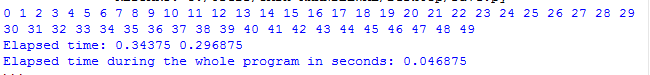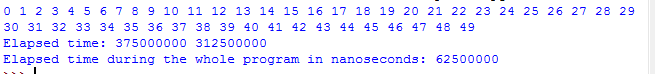Open in App
Not now

# time.process_time() function in Python

• Difficulty Level : Basic
• Last Updated : 18 Apr, 2019

`time.process_time() ` function always returns the float value of time in seconds. Return the value (in fractional seconds) of the sum of the system and user CPU time of the current process. It does not include time elapsed during sleep. The reference point of the returned value is undefined, so that only the difference between the results of consecutive calls is valid.

As time module provides various time-related functions. So it is necessary to import the time module otherwise it will through error because of the definition of `time.process_time()` is present in `time module`.

Example: Understand the usage of the process_time().

 `# Python program to show time by process_time()  ` `from` `time ``import` `process_time ` ` `  `# assigning n = 50  ` `n ``=` `50`  ` `  `# Start the stopwatch / counter  ` `t1_start ``=` `process_time()  ` `  `  `for` `i ``in` `range``(n): ` `    ``print``(i, end ``=``' '``) ` ` `  `print``()  ` ` `  `# Stop the stopwatch / counter ` `t1_stop ``=` `process_time() ` `  `  `print``(``"Elapsed time:"``, t1_stop, t1_start)  ` `  `  `print``(``"Elapsed time during the whole program in seconds:"``, ` `                                         ``t1_stop``-``t1_start)  `

Output:process_time_ns():
It always gives the integer value of time in nanoseconds. Similar to process_time() but return time as nanoseconds.This is only the basic difference.

Example: Understand the usage of the `process_time_ns()`.

 `# Python program to show time by process_time_ns()  ` `from` `time ``import` `process_time_ns ` `  `  `n ``=` `50`  ` `  `# Start the stopwatch / counter ` `t1_start ``=` `process_time_ns()  ` `  `  `for` `i ``in` `range``(n): ` `    ``print``(i, end ``=``' '``)  ` ` `  `print``()  ` ` `  `# Stop the stopwatch / counter ` `t1_stop ``=` `process_time_ns() ` `  `  `print``(``"Elapsed time:"``, t1_stop, t1_start) ` `  `  `print``(``"Elapsed time during the whole program in nanoseconds:"``, ` `                                            ``t1_stop``-``t1_start)  `

Output:Note: `process_time()` is very different from `pref_counter()`, as `perf_counter()` calculates the program time with sleep time and if any interrupt is there but process_counter only calculates the system and the CPU time during the process it not includes the sleep time.